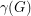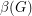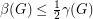# feedback edge set

## Non-edges vs. feedback edge sets in digraphs ★★★

Author(s): Chudnovsky; Seymour; Sullivan

For any simple digraph, we letbe the number of unordered pairs of nonadjacent vertices (i.e. the number of non-edges), andbe the size of the smallest feedback edge set.

Conjecture  Ifis a simple digraph without directed cycles of length, then.

Keywords: acyclic; digraph; feedback edge set; triangle free